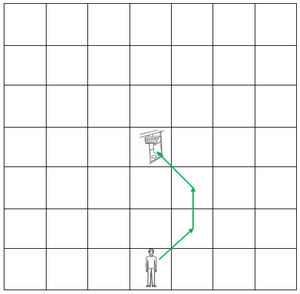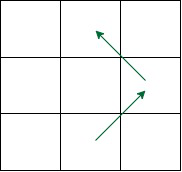Open In App

# Minimize cost to reach a cell in Matrix using horizontal, vertical and diagonal moves

Given two points P1(x1, y1) and P2(x2, y2) of a matrix, the task is to find the minimum cost to reach P2 from P1 when:

• A horizontal or vertical movement in any direction cost 1 unit
• A diagonal movement in any direction costs 0 unit.

Examples:

Input: P1 = {7, 4}, P2 = {4, 4}
Output: 1
Explanation: The movements are given below given below:Movements are (7, 4) -> (6, 5) -> (5, 5) -> (4, 4).
As there is only 1 vertical movement so cost will be 1.

Input: P1  = {1, 2}, P2 = {2, 2}
Output: 1

Approach: The movements should be such that there is minimum horizontal or vertical movement. This can be decided from the following observation:

If the horizontal distance is h = (y2 – y1) and the vertical distance between the points is v = (x2 – x1):
If  |h – v| is even, only diagonal movements are enough.
Because horizontal or vertical positions can be maintained with two opposite diagonal movements as shown in the image below:maintaining vertical position

And when horizontal and vertical distances become same, then can directly move to P2 using diagonal movements.
But in case this value is odd then one vertical or horizontal movement is required to make it even.

For this follow the below steps:

• Find the vertical distance from P1 to P2. Let say it is vert.
• Find the horizontal distance from P1 to P2. Let say it is hori.
• There will always be a way from source to destination by moving diagonally if |hori – vert| is even.
• But if |hori – vert| is odd then 1 step either horizontal or vertical is required after that only diagonal movement will be sufficient.

Below is the implementation of the above approach.

## C++

 `// C++ algorithm for above approach``#include ``using` `namespace` `std;` `// Function to find Minimum Cost``int` `minCostToExit(``int` `x1, ``int` `y1, ``int` `x2, ``int` `y2)``{``    ``// Finding vertical distance``    ``// from destination``    ``int` `vert = ``abs``(x2 - x1);` `    ``// Finding horizontal distance``    ``// from destination``    ``int` `hori = ``abs``(y1 - y2);` `    ``// Finding the minimum cost``    ``if` `(``abs``(hori - vert) % 2 == 0)``        ``return` `0;``    ``else``        ``return` `1;``}` `// Driver code``int` `main()``{``    ``int` `x1 = 7;``    ``int` `y1 = 4;``    ``int` `x2 = 4, y2 = 4;``    ``int` `cost = minCostToExit(x1, y1, x2, y2);``    ``cout << cost;``    ``return` `0;``}`

## Java

 `// Java algorithm for above approach``import` `java.io.*;``class` `GFG {` `  ``// Function to find Minimum Cost``  ``static` `int` `minCostToExit(``int` `x1, ``int` `y1,``                           ``int` `x2, ``int` `y2)``  ``{` `    ``// Finding vertical distance``    ``// from destination``    ``int` `vert = Math.abs(x2 - x1);` `    ``// Finding horizontal distance``    ``// from destination``    ``int` `hori = Math.abs(y1 - y2);` `    ``// Finding the minimum cost``    ``if` `(Math.abs(hori - vert) % ``2` `== ``0``)``      ``return` `0``;``    ``else``      ``return` `1``;``  ``}` `  ``// Driver code``  ``public` `static` `void` `main(String args[]) {``    ``int` `x1 = ``7``;``    ``int` `y1 = ``4``;``    ``int` `x2 = ``4``, y2 = ``4``;``    ``int` `cost = minCostToExit(x1, y1, x2, y2);``    ``System.out.println(cost);``  ``}``}` `// This code is contributed by Saurabh Jaiswal`

## Python3

 `# Python algorithm for above approach``import` `math as Math` `# Function to find Minimum Cost``def` `minCostToExit (x1, y1, x2, y2):` `    ``# Finding vertical distance``    ``# from destination``    ``vert ``=` `Math.fabs(x2 ``-` `x1);` `    ``# Finding horizontal distance``    ``# from destination``    ``hori ``=` `Math.fabs(y1 ``-` `y2);` `    ``# Finding the minimum cost``    ``if` `(Math.fabs(hori ``-` `vert) ``%` `2` `=``=` `0``):``        ``return` `0``;``    ``else``:``        ``return` `1``;` `# Driver code``x1 ``=` `7``y1 ``=` `4``x2 ``=` `4``y2 ``=` `4``;``cost ``=` `minCostToExit(x1, y1, x2, y2);``print``(cost);` `# This code is contributed by gfgking`

## C#

 `// C# algorithm for above approach``using` `System;``class` `GFG {` `  ``// Function to find Minimum Cost``  ``static` `int` `minCostToExit(``int` `x1, ``int` `y1, ``int` `x2, ``int` `y2)``  ``{``    ``// Finding vertical distance``    ``// from destination``    ``int` `vert = Math.Abs(x2 - x1);` `    ``// Finding horizontal distance``    ``// from destination``    ``int` `hori = Math.Abs(y1 - y2);` `    ``// Finding the minimum cost``    ``if` `(Math.Abs(hori - vert) % 2 == 0)``      ``return` `0;``    ``else``      ``return` `1;``  ``}` `  ``// Driver code``  ``public` `static` `void` `Main()``  ``{``    ``int` `x1 = 7;``    ``int` `y1 = 4;``    ``int` `x2 = 4, y2 = 4;``    ``int` `cost = minCostToExit(x1, y1, x2, y2);``    ``Console.Write(cost);``  ``}``}` `// This code is contributed by ukasp.`

## Javascript

 ``

Output

`1`

Time Complexity: O(1)
Auxiliary Space: O(1), since no extra space has been taken.